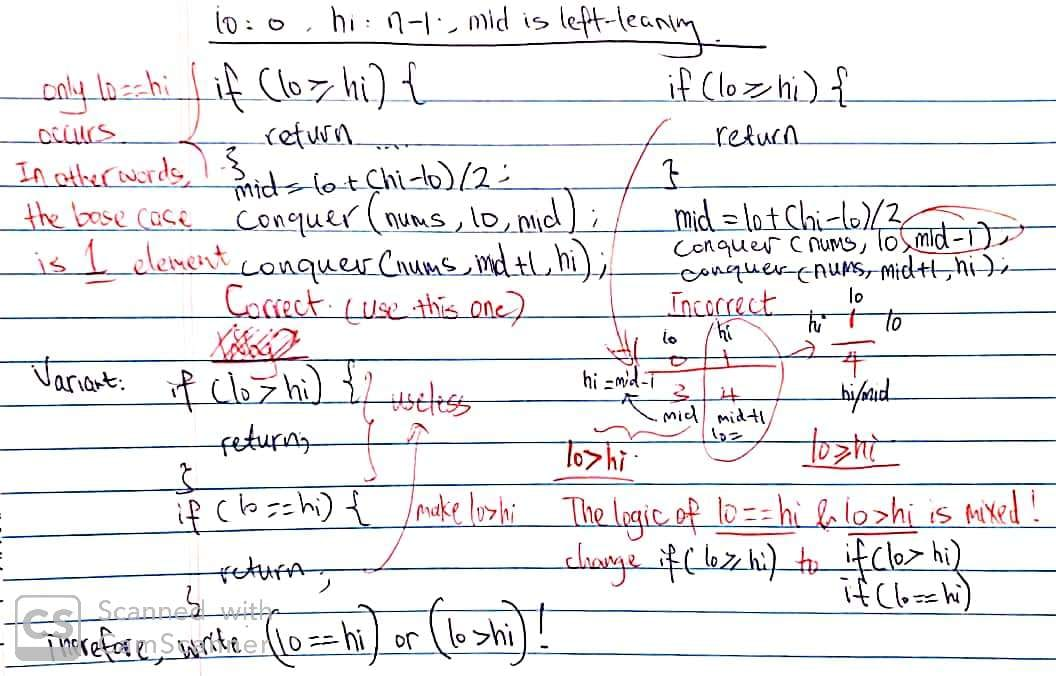# 53. Maximum Subarray

Reference: LeetCode
Difficulty: Easy

## Problem

Given an integer array nums, find the contiguous subarray (containing at least one number) which has the largest sum and return its sum.

Example:

Follow up: If you have figured out the $O(N)$ solution, try coding another solution using the divide and conquer approach, which is more subtle.

## Analysis

### Most Stupid Solution

For each element, we construct all possible subarrays starting from this element. Totally there are at most $N^2$ subarrays. Also, calculating the sum of each subarray takes $O(N)$.

Time: $O(N^3)$
Space: $O(1)$

### Brute-Force

Why did you calculate the sum separately?

Note: In the inner loop, start from i + 1. Don’t initialize sum as $0$ and start from i.

Time: $O(N^2)$
Space: $O(1)$

### Divide and Conquer

Divide-and-conquer consider 3 cases:

• Case 1: Subarray in the left half -> leftSum
• Case 2: Subarray in the right half -> rightSum
• Case 3: Subarray crosses the middle -> crossSum

We need to compare three max values: leftSum, rightSum, and crossSum. By constructing the crossSum, we propagate from the right-end of the left subarray [lo, mid] and from the left-end of the right subarray [mid + 1, hi]. In each direction, we are continuously updating the maximum sum.

BTW, Index lo/hi/mid Caveat:

Write the condition as if (lo == hi) (stops at one element) or if (lo >= hi) (stops at $0$ element). Why?

Write the subproblem as [lo, mid] and [mid + 1, hi], which is not like binary search (hi = mid - 1 and lo = mid + 1).Another version, initialize sums and max values as the first element.

Time: $O(N\log{N})$ since $T(N) = 2T(N/2) + O(N)$.
Space: $O(\log{N})$

### DP

Suppose we know the maximum subarray ending at $i$ (inclusive). We denote SUM(i) as the maximum sum of a subarray ending at index $i$ and denote OPT(i) as the maximum sum in the subarray $[0, i]$. Our final result is OPT(n - 1). (notice the difference since it is very trivial)

For an element nums[i], we have two choices: Appending it to a previous subarray SUM(i - 1) or start a new subarray from itself. Then we can write the recurrence for SUM(i) and OPT(i) as follows:

SUM(i) = max(SUM(i - 1) + nums[i], nums[i])
OPT(i) = max(OPT(i - 1), SUM(i)).

Note: OPT is updated when a larger SUM[i] is discovered.

The initial values are SUM(0) = nums and OPT(0) = nums. We can do it in one pass. So here is the code:

Since SUM(i) and OPT(i) could be calculated by the previous values, we don’t need arrays of size $n$ to store all information. Here is the code that reduces the space complexity:

Time: $O(N)$
Space: $O(1)$

CommentJunhao Wang
a software engineering cat